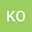A solution formula and the R-boundedness for the generalized Stokes resolvent problem in an infinite layer with Neumann boundary condition
•#### Peer review status:UNDER REVIEW

24 Jun 2020Submitted to Mathematical Methods in the Applied Sciences
27 Jun 2020Assigned to Editor
27 Jun 2020Submission Checks Completed
27 Jun 2020Reviewer(s) Assigned

## Abstract

We consider the generalized Stokes resolvent problem in an infinite layer with Neumann boundary conditions. This problem arises from a free boundary problem describing the motion of incompressible viscous one-phase fluid flow without surface tension in an infinite layer bounded both from above and from below by free surfaces. We derive a new exact solution formula to the generalized Stokes resolvent problem and prove the $\mathscr{R}$-boundedness of the solution operator families with resolvent parameter $\lambda$ varying in a sector $\Sigma_{\varepsilon,\gamma_0}$ for any $\gamma_0>0$ and $0<\varepsilon<\pi/2$, where $\Sigma_{\varepsilon,\gamma_0} =\{ \lambda\in\mathbb{C}\setminus\{0\} \mid |\arg\lambda|\leq\pi-\varepsilon, \ |\lambda|>\gamma_0 \}$. As applications, we obtain the maximal $L_p$-$L_q$ regularity for the nonstationary Stokes problem and then establish the well-posedness locally in time of the nonlinear free boundary problem mentioned above in $L_p$-$L_q$ setting. We make full use of the solution formula to take $\gamma_0>0$ arbitrarily, while in general domains we only know the $\mathscr{R}$-boundedness for $\gamma_0\gg1$ from the result by Shibata. As compared with the case of Neumann-Dirichlet boundary condition studied by Saito, analysis is even harder on account of higher singularity of the symbols in the solution formula.